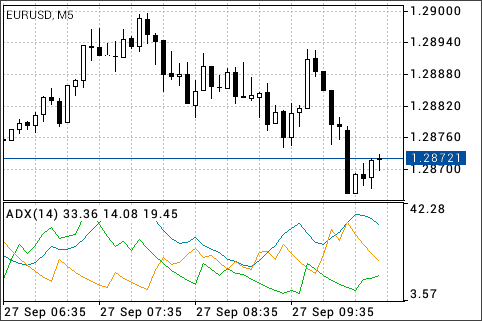MetaTrader 5 Android 帮助图表指标趋势指标平均定向运动指数 (Average Directional Movement Index)

# 平均定向运动指数 (Average Directional Movement Index)## 计算

ADX = SUM ((+DI - (-DI)) / (+DI + (-DI)), N) / N

N ― 计算所用的周期数;
SUM (..., N) ― N 周期的总合;
+DI ― 正向价格走势的指标值 (正向指标);
-DI ― 负向价格走势的指标值 (负向指标)。